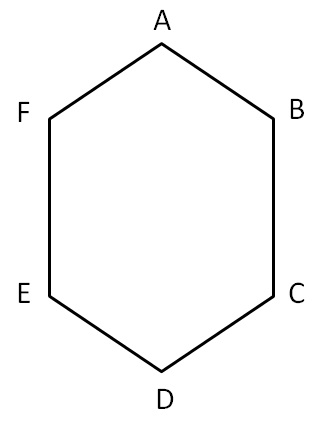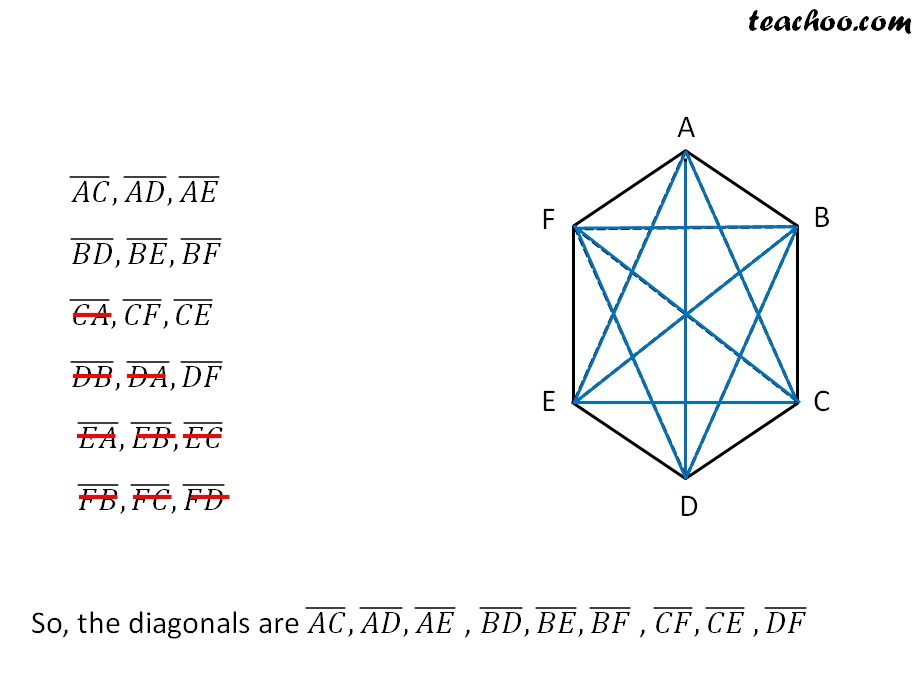Curve, Polygons

Chapter 4 Class 6 Basic Geometrical Ideas
Concept wise

Let it take an example of a polygon.Here, points A, B, C, D, E and F, are the vertices of the polygon.

AB, BC, CD, DE, EF and FA are the sides of the polygon.

## Diagonals of a polygon

Diagonal is the line joining any two vertices which are not adjacent.Learn in your speed, with individual attention - Teachoo Maths 1-on-1 Class

### Transcript

Diagonals of a polygon Diagonal is the line joining any two vertices which are not adjacent. (𝐴𝐶) ̅,(𝐴𝐷) ̅,(𝐴𝐸) ̅ (𝐵𝐷) ̅,(𝐵𝐸) ̅,(𝐵𝐹) ̅ (𝐶𝐴) ̅,(𝐶𝐹) ̅,(𝐶𝐸) ̅ (𝐷𝐵) ̅,(𝐷𝐴) ̅,(𝐷𝐹) ̅ (𝐸𝐴) ̅,(𝐸𝐵) ̅,(𝐸𝐶) ̅ (𝐹𝐵) ̅,(𝐹𝐶) ̅,(𝐹𝐷) ̅ So, the diagonals are (𝐴𝐶) ̅,(𝐴𝐷) ̅,(𝐴𝐸) ̅ , (𝐵𝐷) ̅,(𝐵𝐸) ̅,(𝐵𝐹) ̅ , (𝐶𝐹) ̅,(𝐶𝐸) ̅ ,(𝐷𝐹) ̅